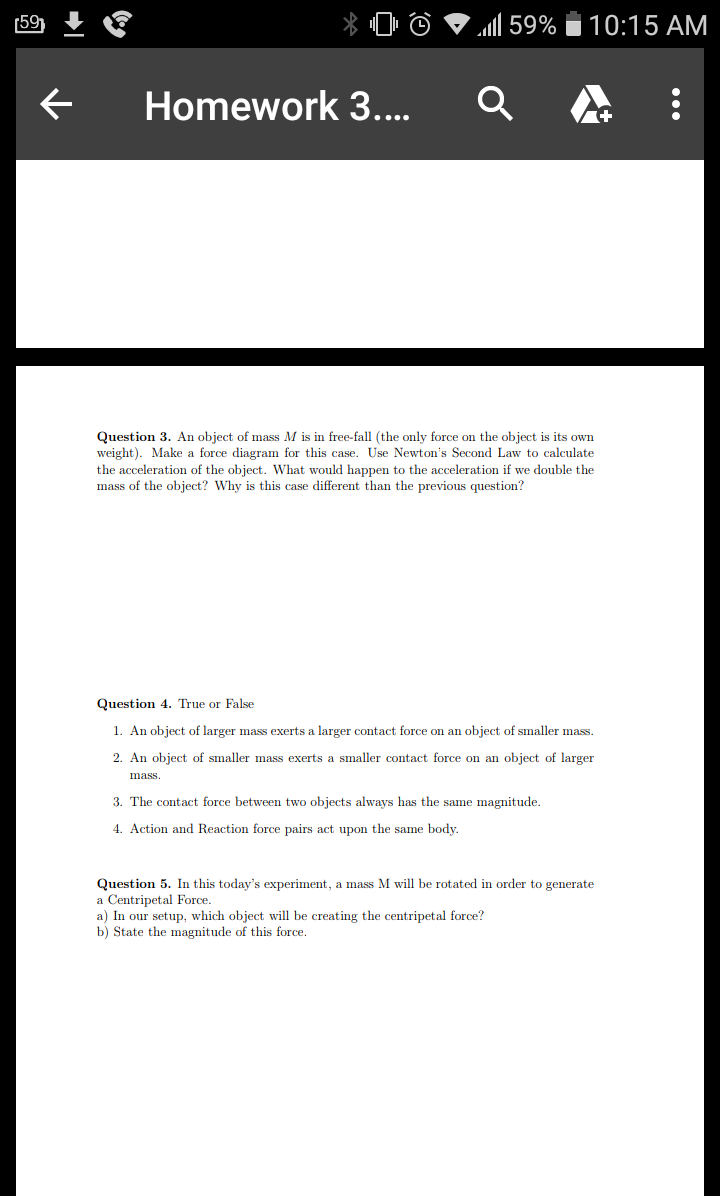159x 0.11 59%10:15 AMHomework 3Question 3. An object of mass M is in free-fall (the only force on the object is its ownweight). Make a force diagram for this case. Use Newton's Second Law to calculatethe acceleration of the object. What would happen to the acceleration if we double themass of the object? Why is this case different than the previous question?Question 4. True or False1. An object of larger mass exerts a larger contact force on an object of smaller mass2. An object of smaller mass exerts a smaller contact force on an object of largermass3. The contact force between two objects always has the same magnitude4. Action and Reaction force pairs act upon the same body.Question 5. In this today's experiment, a mass M will be rotated in order to generatea Centripetal Force.a) In our setup, which object will be creating the centripetal force?b) State the magnitude of this force

Question

Question 5help_outlineImage Transcriptionclose159 x 0 .11 59% 10:15 AM Homework 3 Question 3. An object of mass M is in free-fall (the only force on the object is its own weight). Make a force diagram for this case. Use Newton's Second Law to calculate the acceleration of the object. What would happen to the acceleration if we double the mass of the object? Why is this case different than the previous question? Question 4. True or False 1. An object of larger mass exerts a larger contact force on an object of smaller mass 2. An object of smaller mass exerts a smaller contact force on an object of larger mass 3. The contact force between two objects always has the same magnitude 4. Action and Reaction force pairs act upon the same body. Question 5. In this today's experiment, a mass M will be rotated in order to generate a Centripetal Force. a) In our setup, which object will be creating the centripetal force? b) State the magnitude of this force fullscreen
Step 1

a)

Centripetal force is the result of circular motion of an object. It is directed towards the center of circular motion along the radius of circular path. Here, an object of mass M is rotating. So the mass M is responsible for creating the centripetal force.

Answer: Mass M creates the centripetal force.

Step 2

b)

Centripetal force is directly proportional to the mass of rotating object, square of linear velocity of motion, an...

Want to see the full answer?

See Solution

Want to see this answer and more?

Our solutions are written by experts, many with advanced degrees, and available 24/7

See Solution
Tagged in

Physics